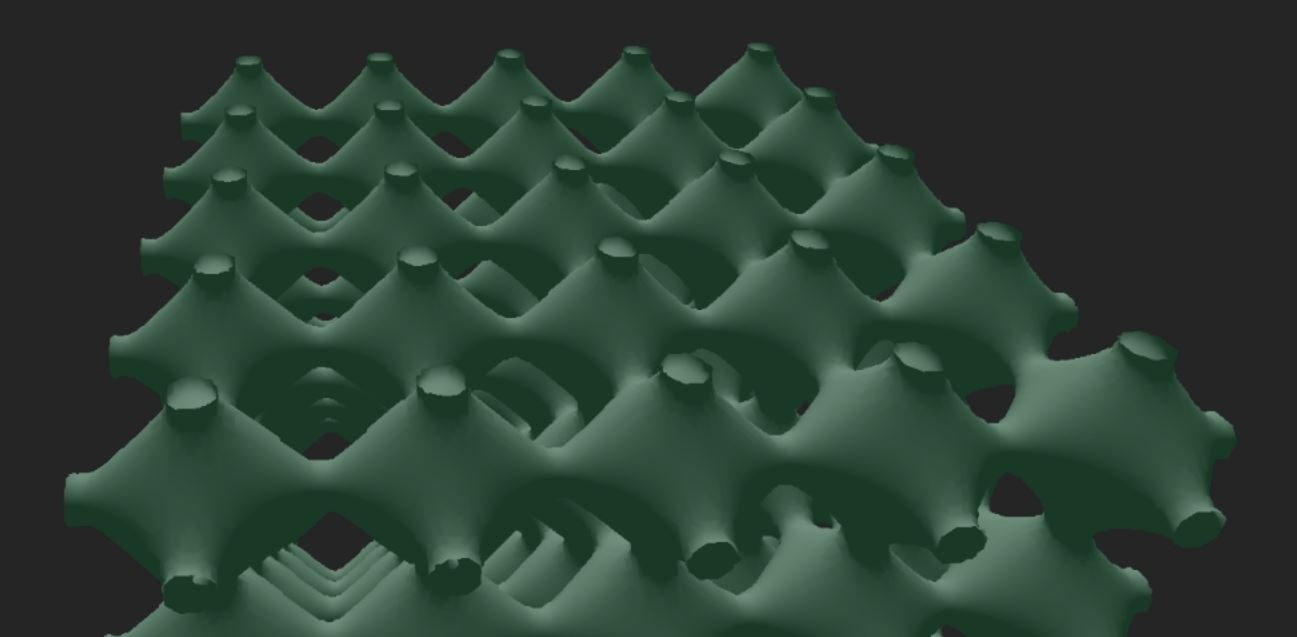# Real Time Marching Cubes

### Goal

Move the calculations for the marching cubes algorithm by Lorensen and Cline to the GPUIn order to explore this I used opengl's geometry shaders and compute shaders

My first attempts to do this involved using cuda and an algorithm known as histopyrimid, however because my use case fit so nicely with the intended use of geometry shaders, I went with that instead. The other problem with the cuda approach was that I would have to copy data back from the cuda section of memory to the cpu, then back to the gpu using OpenGL.

I then decided to use geometry shaders ability to construct arbitrary triangles and render one "point" per cube in the marching cubes algorithm which then created the needed triangles.

I demonstrated the marching cubes part with two different sets of volume data, one generated by formula, one by moving particles.

## Implicit formula

First I did this with an implicit function computed each frame. Recreating the entire volume data via compute shaders and all of the geometry each frame on a 2563 grid. This resulted in between 30 and 60 fps. If I shrunk the grid to 1283 I could get a framerate in excess of 60fps

## Particles

The second way I showed the marching cube algorithm was by simulating objects that leave a trail behind them bouncing around a cube. This demonstraited that the algorithm was applicable to arbitrary volume data, not just an implicit formula